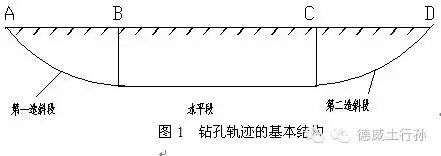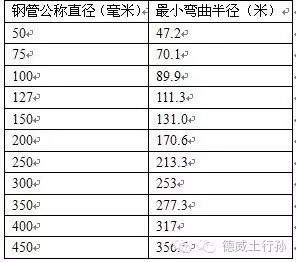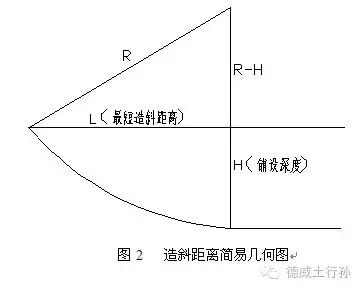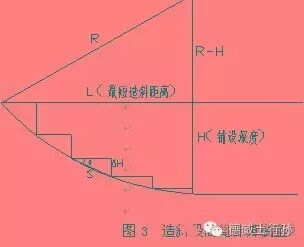# 导向孔设计计算与角度转换2.5 造斜段每根钻杆端点所在的深度、倾角：这是设计计算时最终为操作者需要使用的参数，实际施工时导向人员从导向仪上能读取这些参数，并以此作为导向操作的依据。

3简易设计计算步骤 3.1 计算最短造斜距离L（米） 设产品管线铺设深度（一般由工程发包方给出并考虑地面、地下障碍物及地层条件以后确定的）为H（米），产品管线（或所用钻杆）的最小转弯半径为R（米），则第一造斜段的最短造斜距离L为： L=[R2 -（R-H）2]1/23.2造斜段所用钻杆的根数n（根） 设所用钻杆单根长度为S（米），则造斜段所需使用钻杆的大概根数为： n = L/S （根）4计算举例 某工程铺设PE管，铺设深度3米，使用沟神JT27M1施工，已知钻杆单根长度3米，最小转弯半径54米，设计该工程钻孔轨迹。 解：（1）所需最小造斜距离（即开孔点位置） 根据前述最小造斜距离计算公式，这里R=54米，H=3米，最短造斜距离为 L=[542-（54-3）2 ]1/2=17.75（米） 按前面讨论的轨迹弧线和投影直线间误差问题，这里将计算出的最短造斜距离修正为18米，即钻机需定位在距离水平段起点至少18米或以远。 （2）造斜段所需钻杆最少根数n 根据公式，已知L=18，钻杆单根长3米，故该造斜段所需钻杆至少为n = 18/3 = 6（根） 在（1）、（2）的计算中，也可以先不修正L，直接用计算出的L算出钻杆根数n的值, 再将算出的n值修正成整（根）数。 （3）开孔角计算 由前面列表可知JT27M1钻机的钻杆倾角最大允许改变量为5%，反推法粗算，倾角改变量取最大值，轨迹水平段起点（倾角为0）开始倒数第一根钻杆起点处倾角即为5%， 根据前述公式，该钻杆长度内轨迹深度改变量为 ΔH=S ×tgɑ=3×0.05=0.15（米） 该杆起点处深度值为 H-ΔH =3-0.15=2.85（米）； 以此类推，每相邻两根钻杆倾角变化按最大值5%递增，则各杆起点处的倾角、深度改变量及相应深度值分别为：

5钢管铺设时钻杆倾角最大允许改变量的计算 在产品管线最小转弯半径大于钻杆的最小转弯半径时（例如钢管），每根钻杆倾角最大改变量的计算方法如下： 设该产品管线最小转弯半径为R’，钻杆单根长度为S，则单杆长度内钻孔允许改变的角度大小ɑ为： ɑ= (180/πR’)×S 相应倾角改变量δ为： δ= tgɑ×100%

## 非开挖导向设计基本原理及角度转换如上图所示，一般由以下五段组成:

Rmin≥1000D~1500D

R=57.3/i

i=57.3/R

R：曲率半径；单位：m

i：  造斜强度；单位：°/m

D：钢管外径；单位：mm

φ219mm钢管曲率半径

R=1000D

=0.219X1000

=219m

i =57.3/R

=57.3/219

=0.262(°/m ）

R=1500D.R=1000D.1 保证钻进曲线的曲率半径大于钻杆的最小曲率半径；

2 使曲线的曲率半径尽量大，每米钻杆角度变化尽量小，即造斜强度尽可能小。tgα =H/0.5L

α =H/0.5L=6/51=0.118，

2α=2X11.8%=23.6%

23.6%÷（51-3）=0.492%

H1=3×23.6%=0.708m；

H2=H1+3×22.1%=1.37m；

H3= H2+3×20.6%=1.99m；

H4= H3+3×19.2%=2.56m；

H5= H4+3×17.7%=3.09m；

H6= H5+3×16.2%=3.58m；

H7= H6+3×14.75%=4.02m；

H8= H7+3×13.3%=4.42m；

H9= H8+3×11.8%=4.77m；

H10= H9+3×10.3%=5.08m；

H11=H10+3×8.85%=5.34m；

H12=H11+3×7.37%=5.56m；

H13=H12+3×5.9%=5.73m；

H14=H13+3×4.43%=5.86m；

H15=H14+3×2.95%=5.95m；

H16=H15+3×1.475%=5.99m；

H17=H16+3×1.475%=6m；H0=1.0m

H1=H0+3×30%=1.9m；

H2= H1+3×25%=2.65m；

H3= H2+3×20%=3.25m；

H4= H3+3×15%=3.7m；

H5= H4+3×10%=4.0m；

H6= H5+3×5%=4.15m；

H7= H6+3×(5-0)%=4.3m；

H00= H7=4.3 m

H11= H00-3×5%=4.15m；

H22= H11-3×10%=3.85m；

H33= H22-3×15%=3.4m；

H44= H33-3×20%=2.8m；

H55= H44-3×25%=2.15m；

H66= H55-3×30%=1.25m；3 进行导向计算时，可用角度，也可用百分比。下面两表为百分比与角度之间的换算